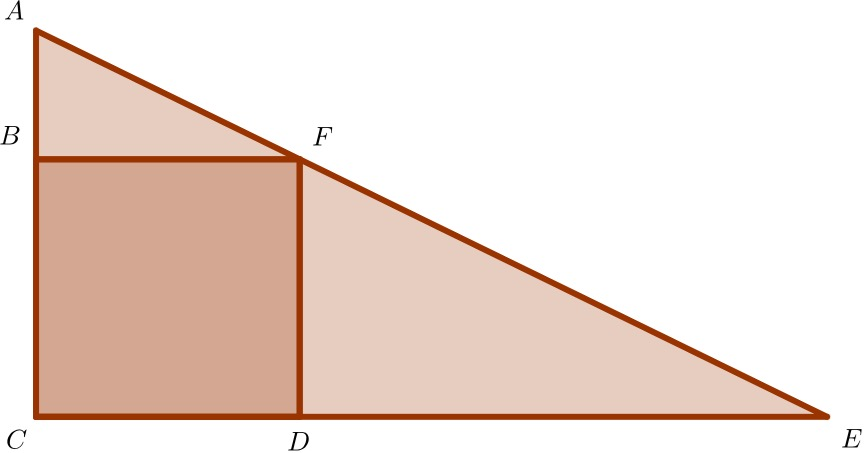# Square and Triangle

Geometry Level 2The above figure is a Triangle of a Triangular Prisms with $BCDF$ Square attached inside.

Given that Area $ABF$ is $25$ and $AB : BF = 1 : 2$ and the height of the Triangular Prisms is $15$

Find the surface Area of the Triangular Prisms